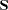The ENTROPY Procedure

### DATA= Data Set

The DATA= data set specified in the PROC ENTROPY statement is the data set that contains the data to be analyzed.

### PDATA= Data Set

The PDATA= data set specified in the PROC ENTROPY statement specifies the support points and prior probabilities to be used in the estimation. The PDATA= can be used in lieu of a PRIORS statement, but is intended for use in conjunction with the OUTP= option. Once priors are entered through a PRIORS statement, they can be reused in subsequent estimations by specifying the PDATA= option.

The variables in the data set are as follows:

• BY variables (if any)

• _TYPE_, a character variable of length 8 that identifies the estimation method: GME or GMENM. This is an optional column.

• variable, a character variable of length 32 that indicates the name of the regressor. The regressor name and the equation name identify a unique coefficient. This is required.

• _OBS_, a numeric variable that is either missing when the probabilities are for coefficients or the observation number when the probabilities are for the residual terms. The _OBS_ and the equation name identify which residual the probability is associated with. This an optional column.

• equation, a character variable of length 32 indicating the name of the dependent variable. This is a required column.

• NSupport, a numeric variable that indicates the number of support points for each basis. This variable is required.

• support, a numeric variable that is the support value the probability is associated with. This is a required column.

• prior, a numeric variable that is the prior probability associated with the probability. This is a required column.

• Prb, a numeric variable that is the estimated probability. This is optional.

### SDATA= Data Set

The SDATA= data set specifies a data set that provides the covariance matrix of the equation errors. The matrix read from the SDATA= data set is used for the equation covariance matrix (matrix) in the estimation. (The SDATA=matrix is used to provide only the initial estimate offor the methods that iterate thematrix.)

Note: This procedure is experimental.Previous Page | Next Page | Top of Page